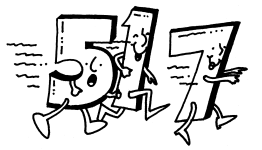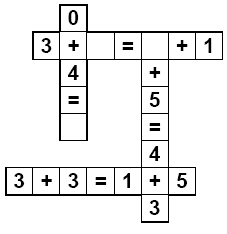Worksheets and No Prep Teaching Resources Runaway Math UnitSecond GradeRunaway Math

Runaway Math Puzzles
Second grade runaway math puzzles
Third grade runaway math puzzles
Fourth grade runaway math puzzles
Fifth and sixth grade runaway math puzzles

Create a custom Runaway Math Puzzle
Create a custom runaway math puzzle
Create a custom runaway math puzzle with missing equations
Create a custom runaway math puzzle where everything is missing

Runaway Math Puzzles: Second GradeAddition - complete the equation (no critical thinking)
Up to three equations with one missing number
All of the equations with one missing number
Addition - Two Missing Numbers from Equations
Two equations are each missing at least two numbers
All of the equations are each missing at least two numbers (not easy)
Addition - Missing All the Numbers in Equations
One equation missing (only numbers)
Two equations missing (only numbers)
Three equations missing (only numbers)
Addition - Missing Entire Equations
One equation missing
Two equations missing
Three equations missing
Addition - Missing Pieces (individual number pieces only)
Three pieces missing
Five pieces missing
Seven pieces missing
Addition - Missing Pieces (may be a number or the symbols: + or =)
Three pieces missing
Five pieces missing
Seven pieces missing

Subtraction - complete the equation (no critical thinking)
Up to three equations with one missing number
All of the equations with one missing number
Subtraction - Two Missing Numbers from Equations
Two equations are each missing at least two numbers
All of the equations are each missing at least two numbers (not easy)
Subtraction - Missing All the Numbers in Equations
One equation missing (only numbers)
Two equations missing (only numbers)
Three equations missing (only numbers)
Subtraction - Missing Entire Equations
One equation missing
Two equations missing
Three equations missing
Subtraction - Missing Pieces (individual number pieces only)
Three pieces missing
Five pieces missing
Seven pieces missing
Subtraction - Missing Pieces (may be a number or the symbols: - or =)
Three pieces missing
Five pieces missing
Seven pieces missing

Addition and Subtraction - fill in the missing operations (no critical thinking)
All of the operations are missing
All of the operations are missing (larger)
Addition and Subtraction - complete the equation (no critical thinking)
Up to three equations with one missing number
All of the equations with one missing number
Addition and Subtraction - Two Missing Numbers from Equations
Two equations are each missing at least two numbers
All of the equations are each missing at least two numbers (not easy)
Addition and Subtraction - Missing All the Numbers in Equations
One equation missing (only numbers)
Two equations missing (only numbers)
Three equations missing (only numbers)
Addition and Subtraction - Missing Entire Equations
One equation missing
Two equations missing
Three equations missing
Addition and Subtraction - Missing Pieces (individual number pieces only)
Three pieces missing
Five pieces missing
Seven pieces missing
Addition and Subtraction - Missing Pieces (may be a number or the symbols: +, -, or =)
Three pieces missing
Five pieces missing
Seven pieces missing

Have a suggestion or would like to leave feedback?